Chapter 27 Electric Fields The electric field of

• Slides: 52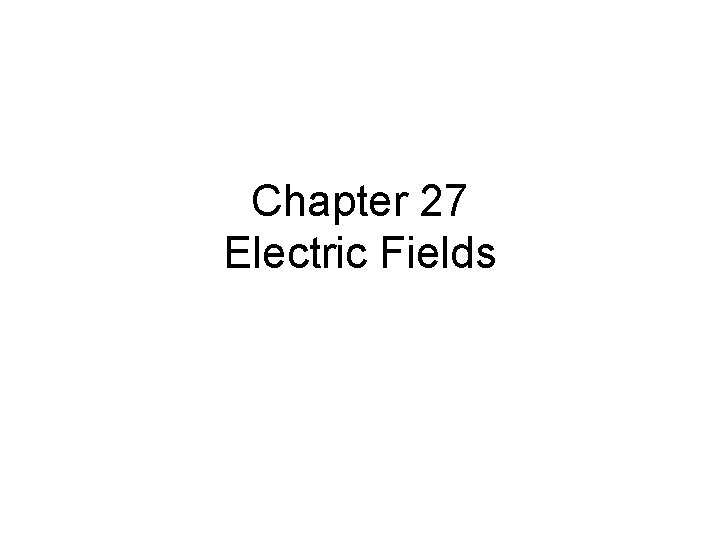Chapter 27 Electric Fields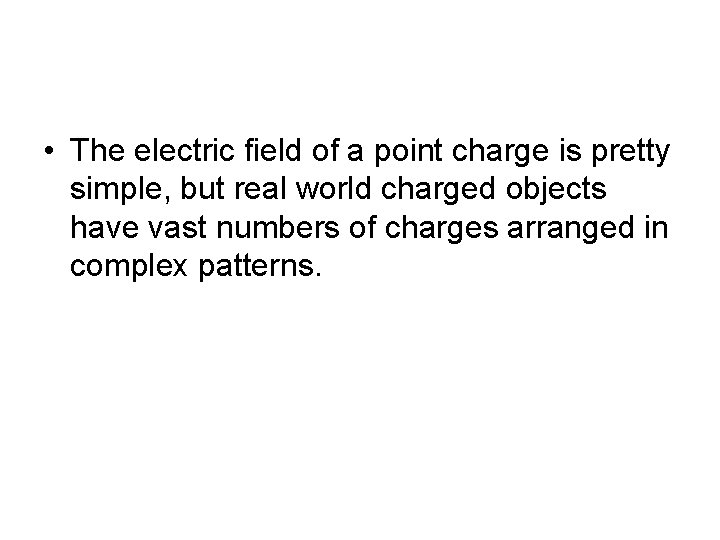• The electric field of a point charge is pretty simple, but real world charged objects have vast numbers of charges arranged in complex patterns.Notice that no matter the size or shape of the charged object, charges seem to space themselves evenly on the surface.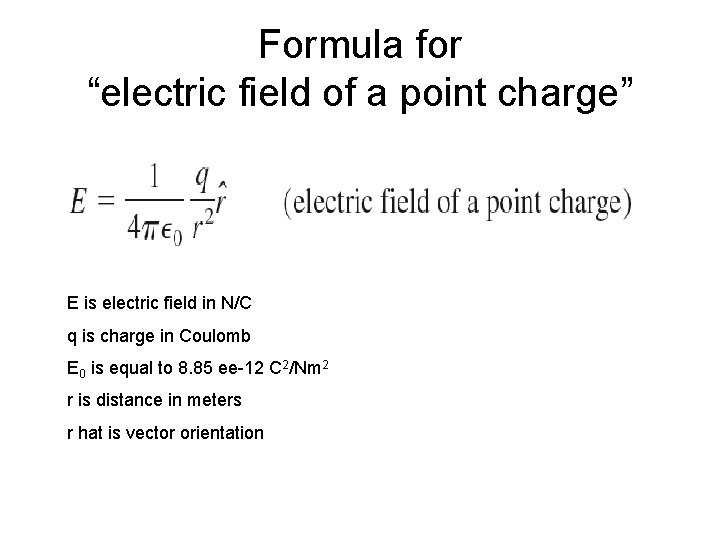Formula for “electric field of a point charge” E is electric field in N/C q is charge in Coulomb E 0 is equal to 8. 85 ee-12 C 2/Nm 2 r is distance in meters r hat is vector orientation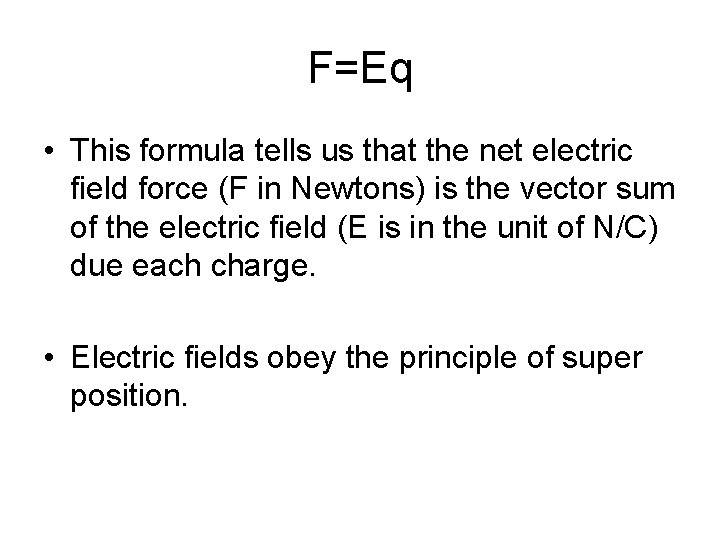F=Eq • This formula tells us that the net electric field force (F in Newtons) is the vector sum of the electric field (E is in the unit of N/C) due each charge. • Electric fields obey the principle of super position.Net electric fields are the result of vector addition.Strategic steps for calculating electric field of multiple points: 1. Establish an x-y system 2. Identify the point charge introduced to the field P 3. Draw in the electric field of each charge on P 4. Use symmetry to determine if the E is zero in any dimension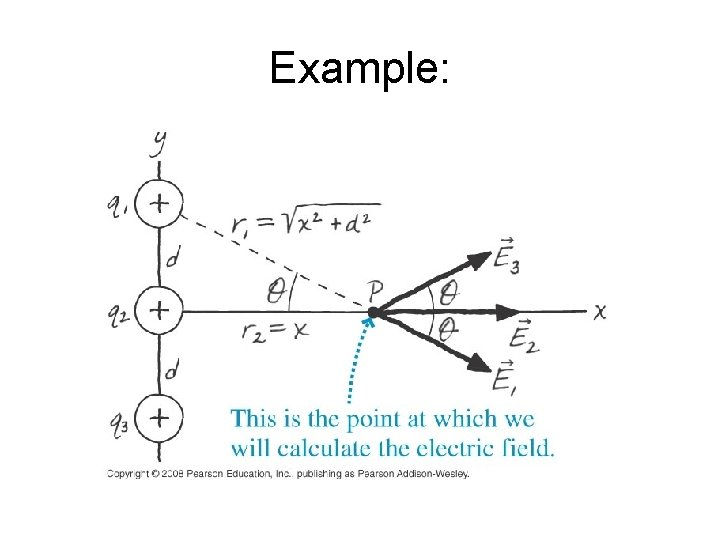Example: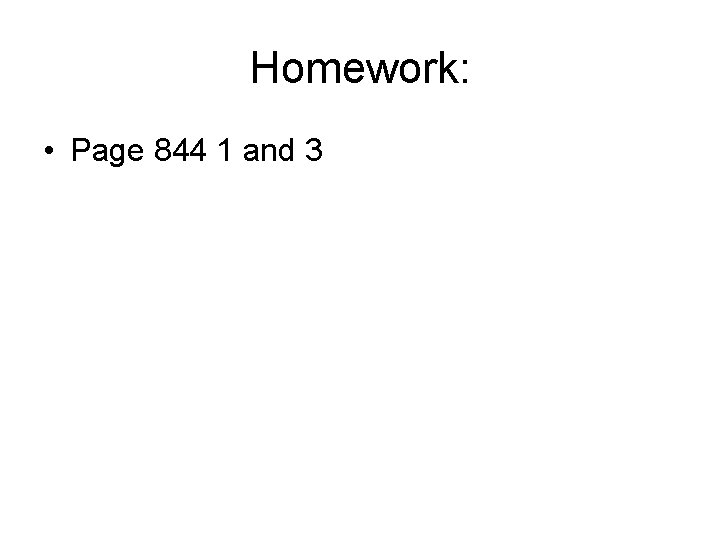Homework: • Page 844 1 and 3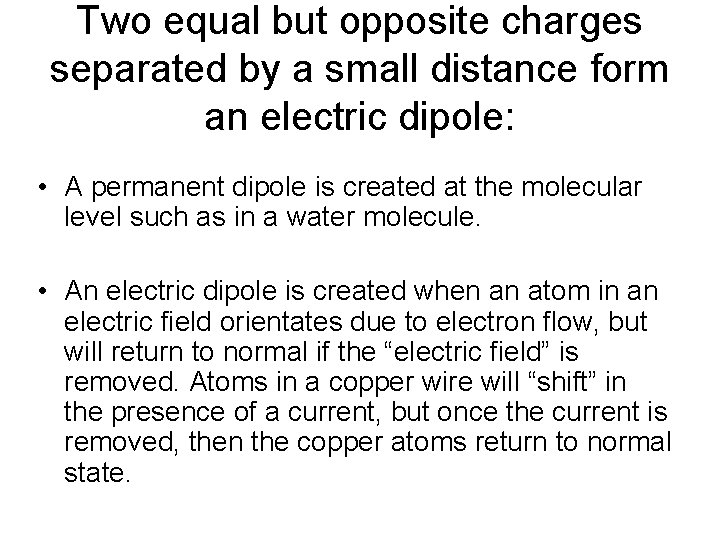Two equal but opposite charges separated by a small distance form an electric dipole: • A permanent dipole is created at the molecular level such as in a water molecule. • An electric dipole is created when an atom in an electric field orientates due to electron flow, but will return to normal if the “electric field” is removed. Atoms in a copper wire will “shift” in the presence of a current, but once the current is removed, then the copper atoms return to normal state.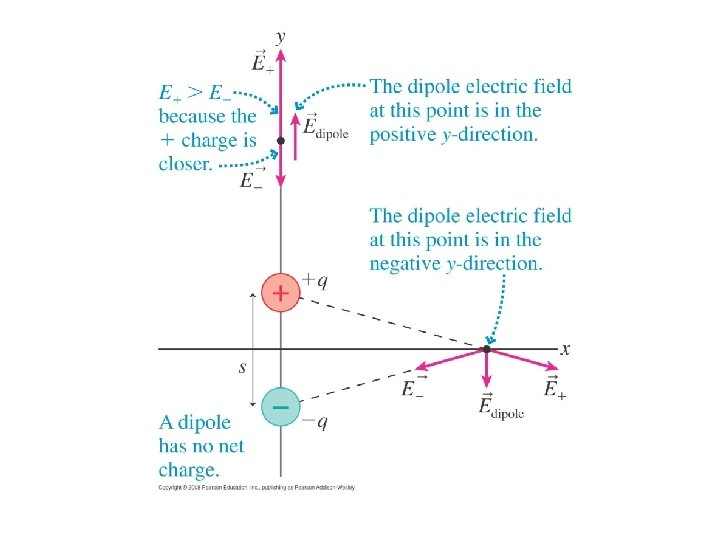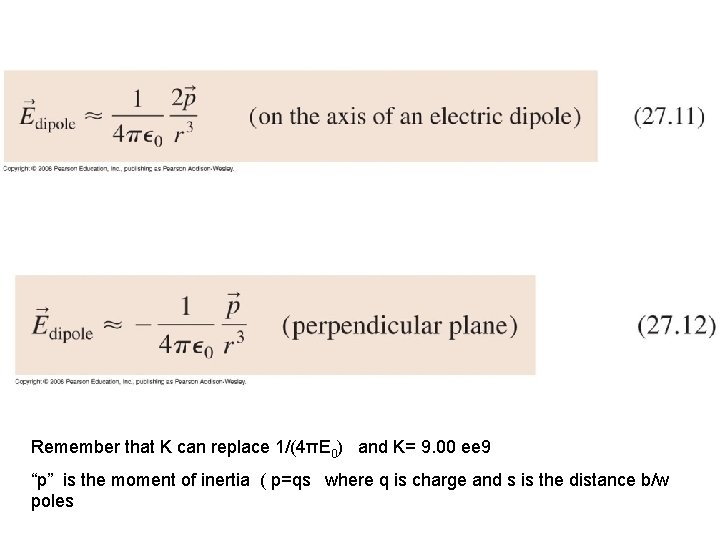Remember that K can replace 1/(4πE 0) and K= 9. 00 ee 9 “p” is the moment of inertia ( p=qs where q is charge and s is the distance b/w poles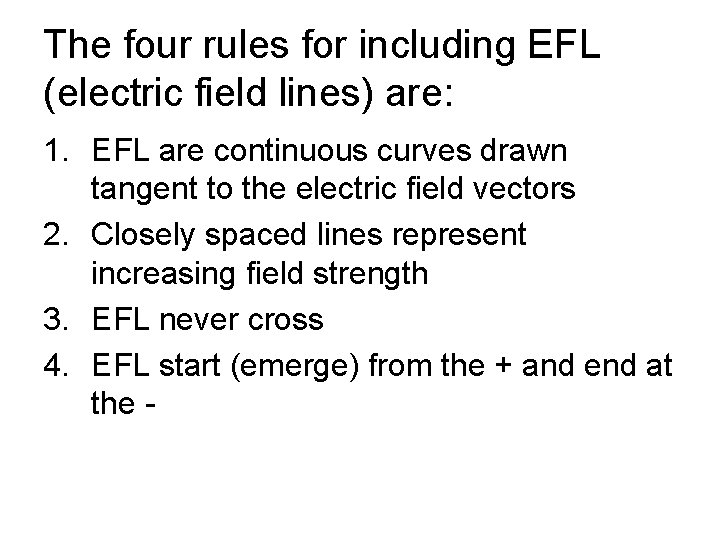The four rules for including EFL (electric field lines) are: 1. EFL are continuous curves drawn tangent to the electric field vectors 2. Closely spaced lines represent increasing field strength 3. EFL never cross 4. EFL start (emerge) from the + and end at the -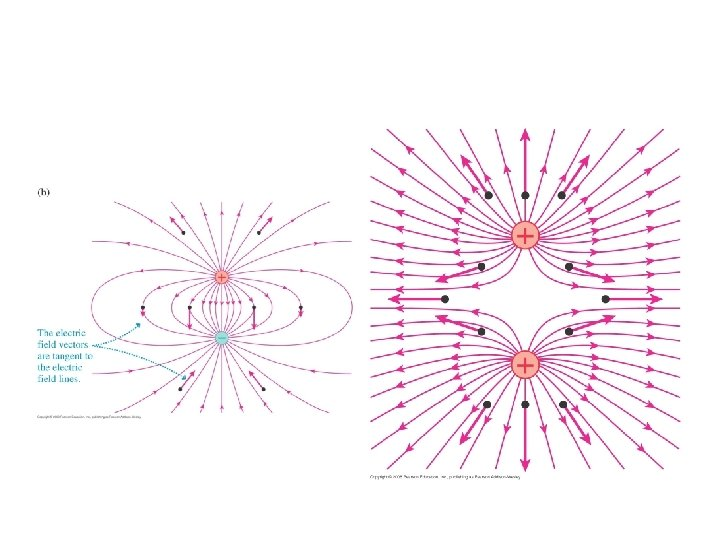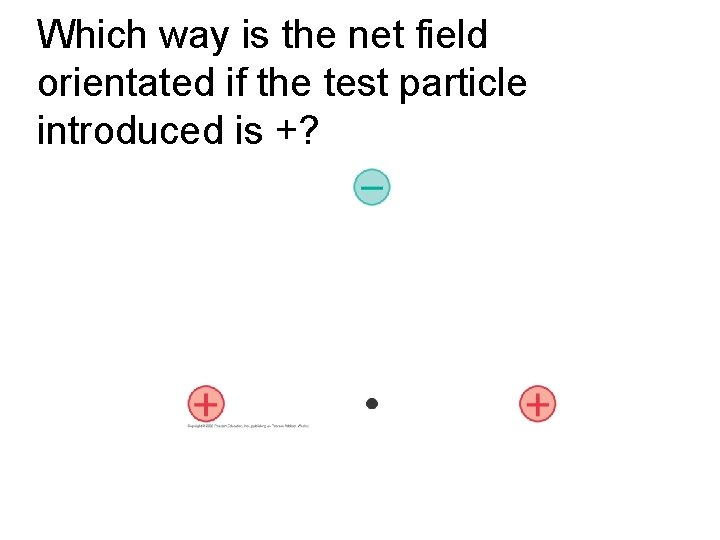Which way is the net field orientated if the test particle introduced is +?• If a charged object contains a large number of excess electrons it is not practical to track every electron…so the charge will be considered continuous and evenly distributed. This distribution is call charge density and varies based on geometry of the charges surface.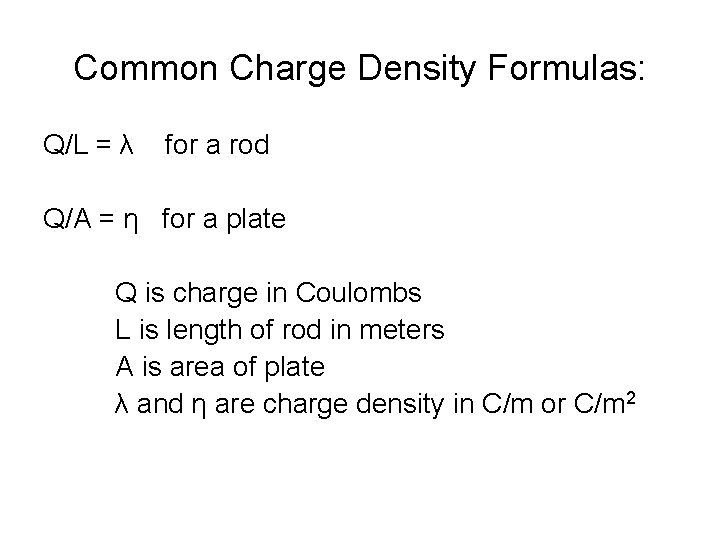Common Charge Density Formulas: Q/L = λ for a rod Q/A = η for a plate Q is charge in Coulombs L is length of rod in meters A is area of plate λ and η are charge density in C/m or C/m 2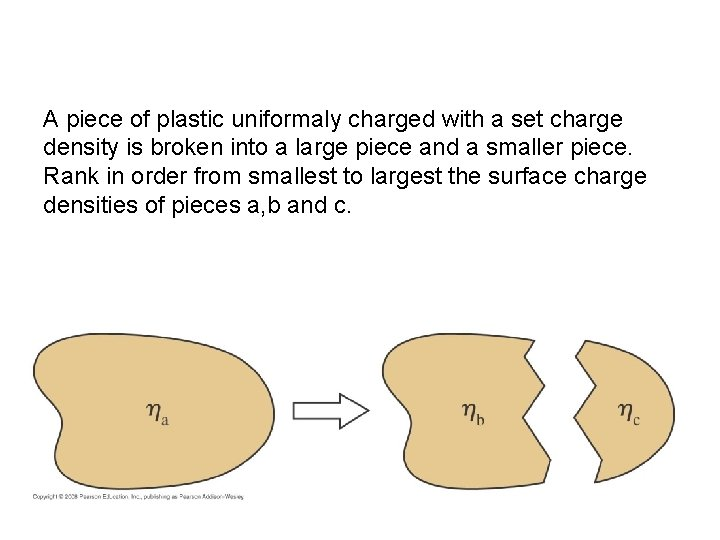A piece of plastic uniformaly charged with a set charge density is broken into a large piece and a smaller piece. Rank in order from smallest to largest the surface charge densities of pieces a, b and c.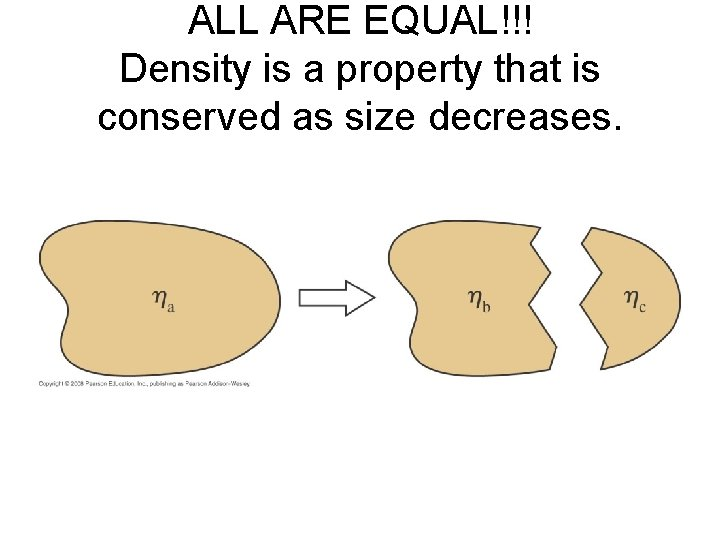ALL ARE EQUAL!!! Density is a property that is conserved as size decreases.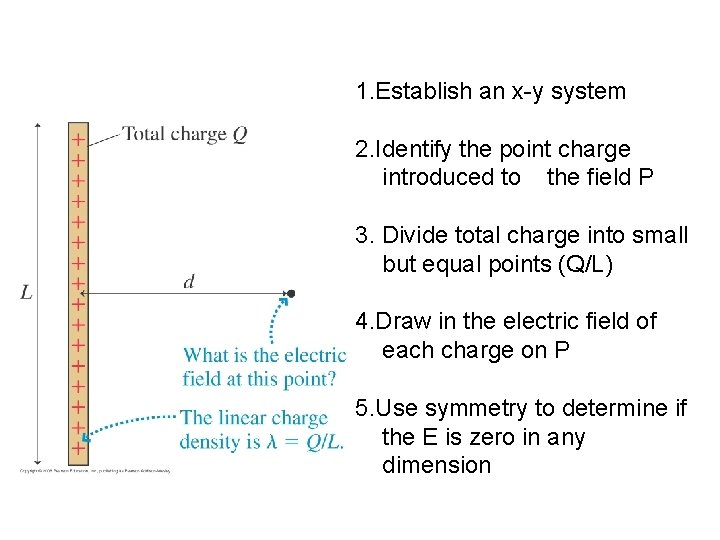1. Establish an x-y system 2. Identify the point charge introduced to the field P 3. Divide total charge into small but equal points (Q/L) 4. Draw in the electric field of each charge on P 5. Use symmetry to determine if the E is zero in any dimensionErod= KQ/ (d√(d 2 + (0. 5 L)2)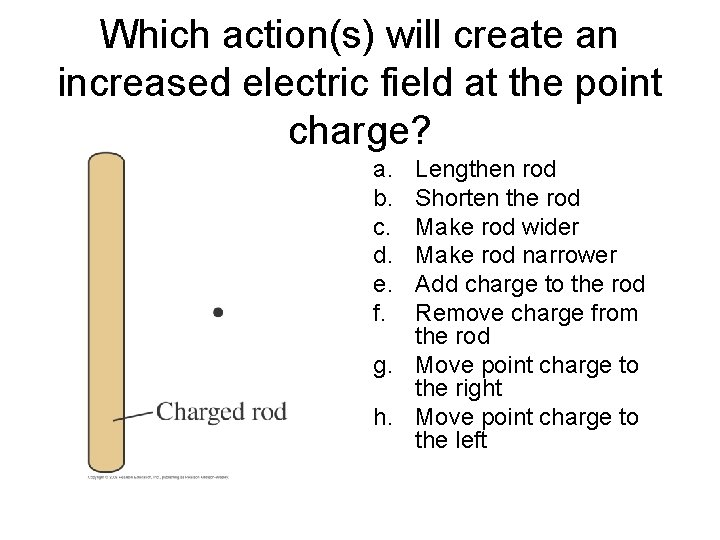Which action(s) will create an increased electric field at the point charge? a. b. c. d. e. f. Lengthen rod Shorten the rod Make rod wider Make rod narrower Add charge to the rod Remove charge from the rod g. Move point charge to the right h. Move point charge to the leftB, E and H work!!! • B concentrated charge when the rod is shortened • E the charge density increases per area • H has a high E due to proximityHomework: • Page 844 -845 6, 7, 8 and 10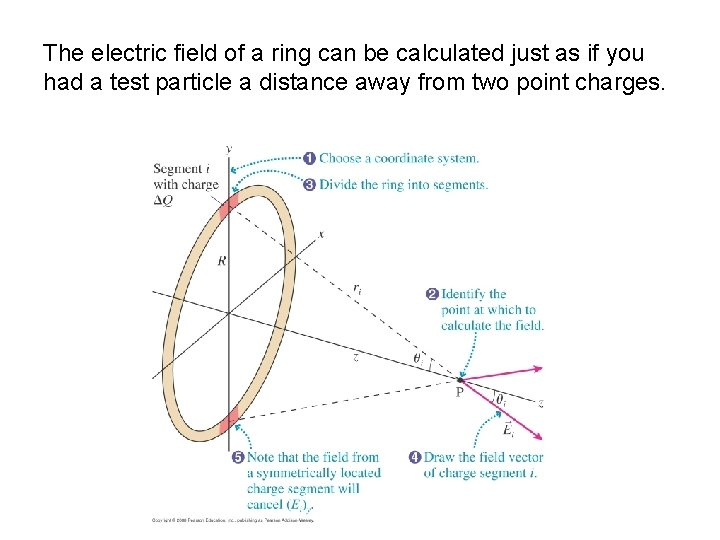The electric field of a ring can be calculated just as if you had a test particle a distance away from two point charges.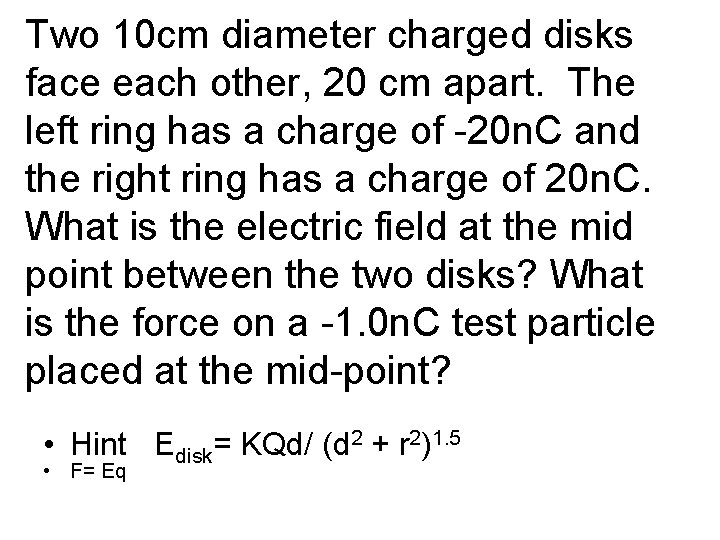Two 10 cm diameter charged disks face each other, 20 cm apart. The left ring has a charge of -20 n. C and the right ring has a charge of 20 n. C. What is the electric field at the mid point between the two disks? What is the force on a -1. 0 n. C test particle placed at the mid-point? • Hint Edisk= KQd/ (d 2 + r 2)1. 5 • F= Eq• There is no electric field at the center of a charged ring because all of the forces cancel!!!• A charged disk is just a series of rings. • The surface charge is spread evenly throughout the surface area of the object. Q/A or Q/πr 2 = η Electric field of a disk can be calculated through KQd/ (d 2 + r 2)1. 5 or (η/2Є0)(1 -(d/√d 2 + r 2)) Є0= 8. 85 ee-12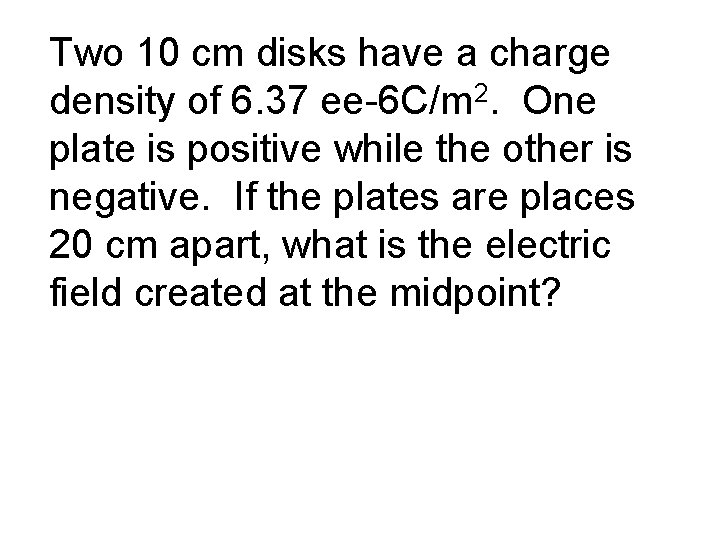Two 10 cm disks have a charge density of 6. 37 ee-6 C/m 2. One plate is positive while the other is negative. If the plates are places 20 cm apart, what is the electric field created at the midpoint?• Homework page 845 # 17, 19 and 21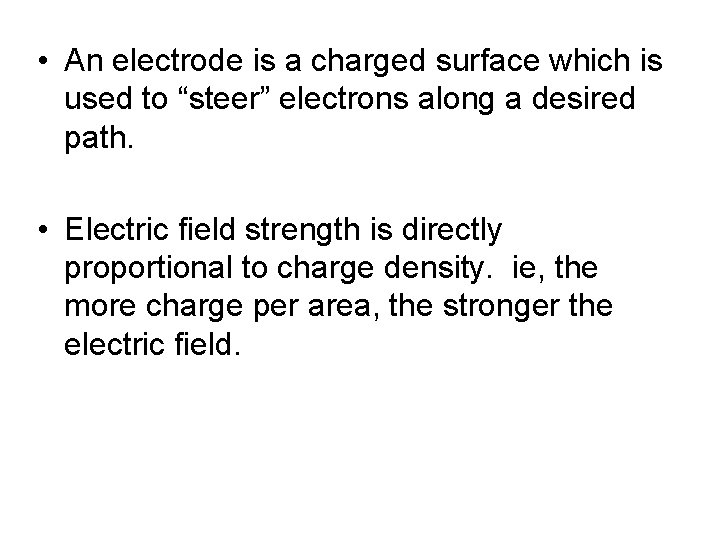• An electrode is a charged surface which is used to “steer” electrons along a desired path. • Electric field strength is directly proportional to charge density. ie, the more charge per area, the stronger the electric field.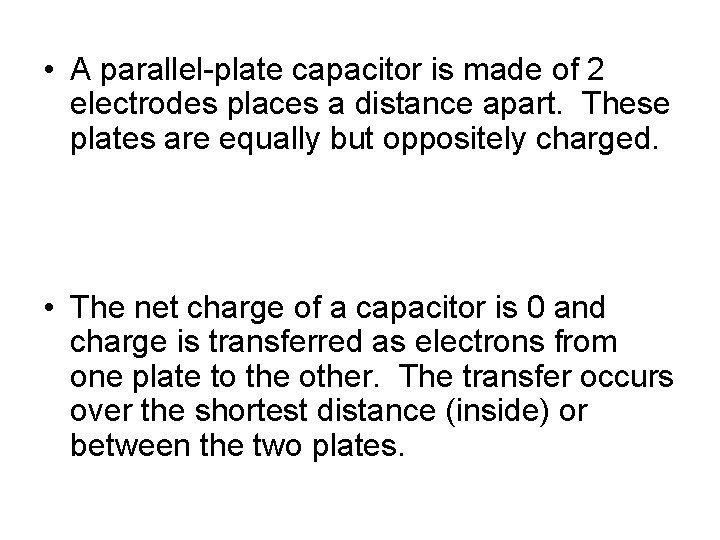• A parallel-plate capacitor is made of 2 electrodes places a distance apart. These plates are equally but oppositely charged. • The net charge of a capacitor is 0 and charge is transferred as electrons from one plate to the other. The transfer occurs over the shortest distance (inside) or between the two plates.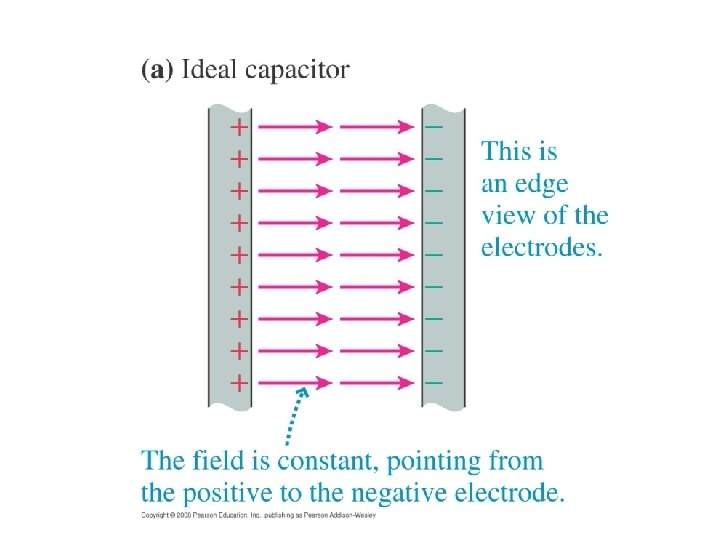Electric field within the capacitor… • Ecap = E+ + E- = η/Є0 = Q/A Є0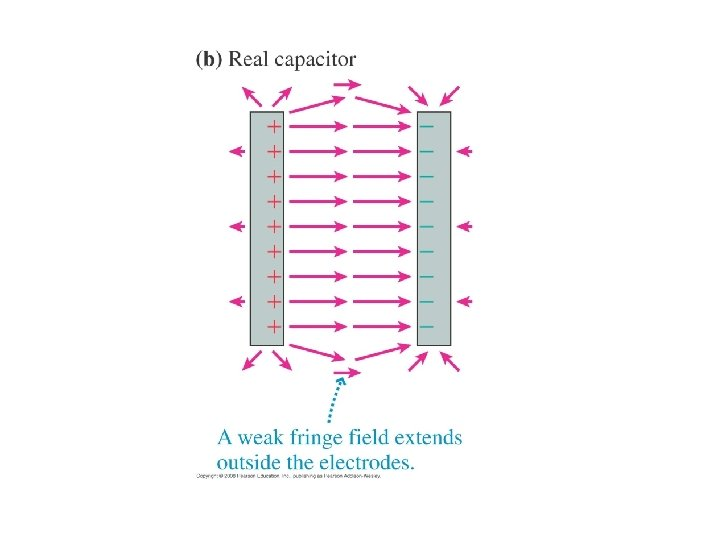• The shape of the electrodes in a capacitor DOES NOT matter!!! As long as the electrodes are close together.At which point would an electron feel the greatest force?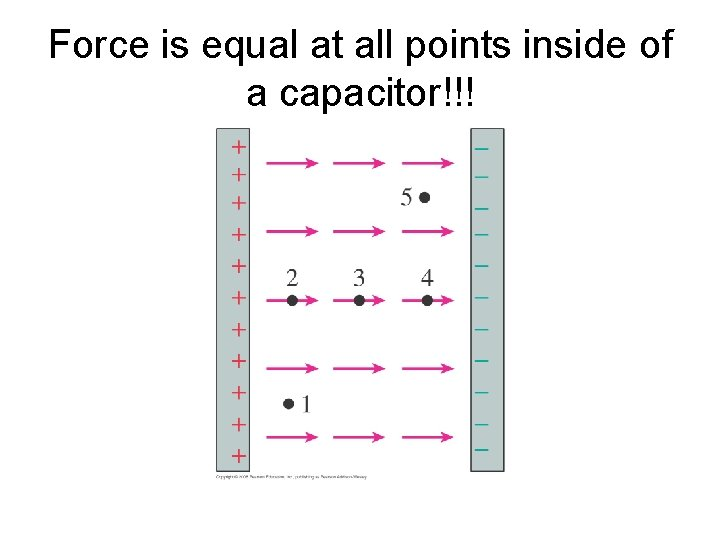Force is equal at all points inside of a capacitor!!!• According to the equation F=q. E, a particle entering an electric field (capacitor) will accelerate (F=ma so ma=q. E). • If the particle is + it accelerates toward the – plate of the capacitor, if the particle is – it will accelerate towards the positive plate of the capacitor. Remember that the electric field in a capacitor always…always points from the positive plate to the negative plate!!!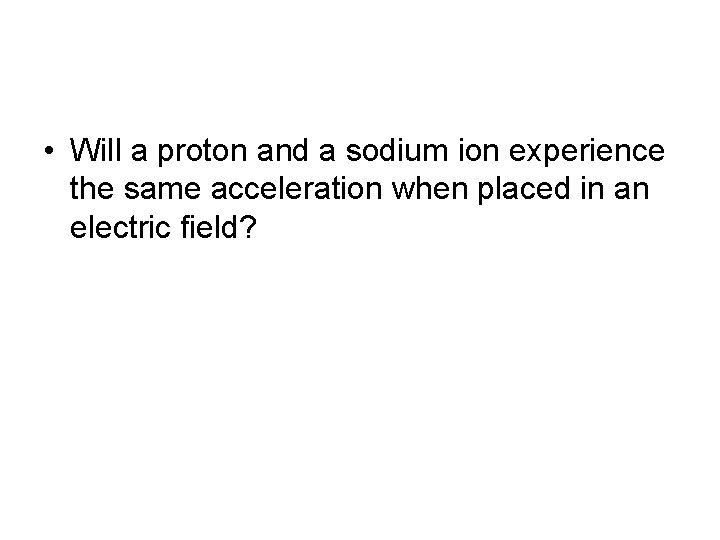• Will a proton and a sodium ion experience the same acceleration when placed in an electric field?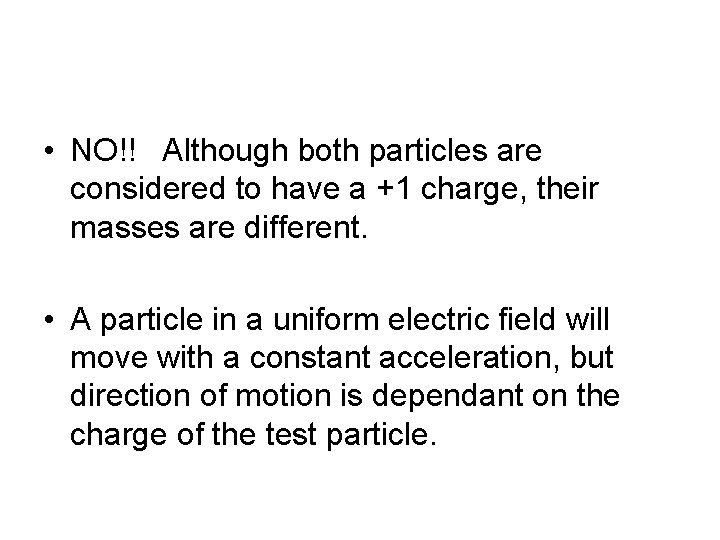• NO!! Although both particles are considered to have a +1 charge, their masses are different. • A particle in a uniform electric field will move with a constant acceleration, but direction of motion is dependant on the charge of the test particle.Uses for particle acceleration in a capacitor: • Old school T. V. screen were the electrons excited the phosphorus covered screen. • Oscilloscopes • Flash on disposable camera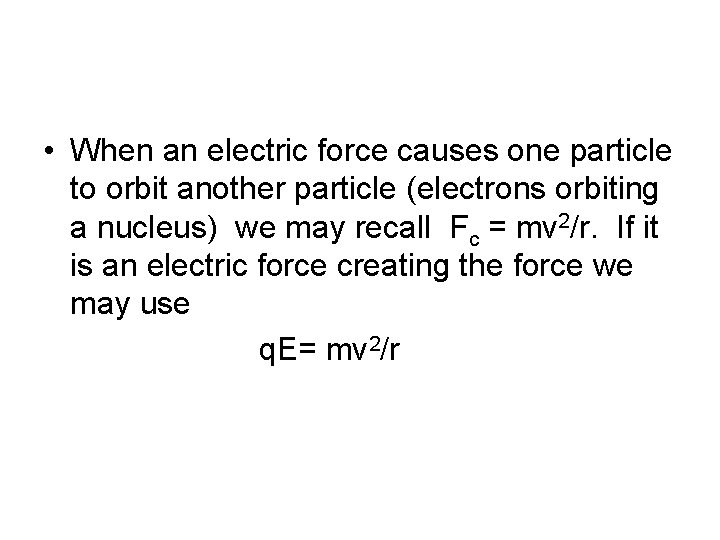• When an electric force causes one particle to orbit another particle (electrons orbiting a nucleus) we may recall Fc = mv 2/r. If it is an electric force creating the force we may use q. E= mv 2/rWhich electric field is responsible for the proton’s trajectory?• B and E are out right away because E lines cannot cross. • A and D are out because a proton moves in the direction of the E lines • C is the best choice!!!If a dipole is placed into an electric field, the dipole will rotate until the net torque it “feels” is zero.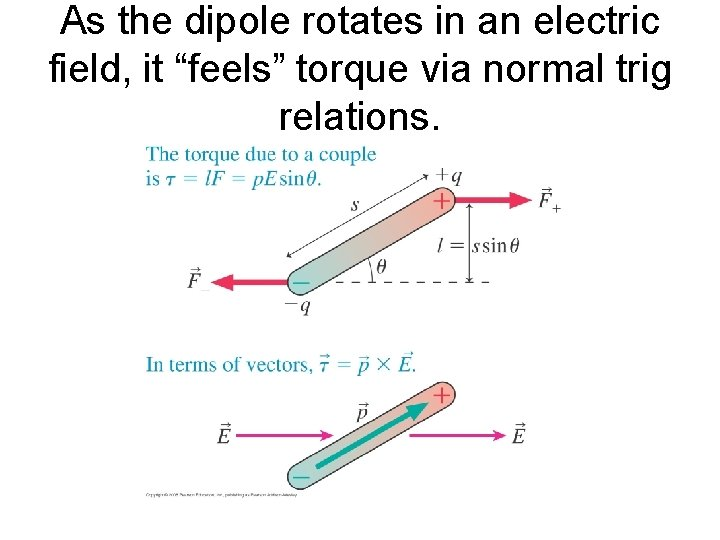As the dipole rotates in an electric field, it “feels” torque via normal trig relations.If a dipole encounters a point charge, the dipole will “flip” in order to align itself with the strongest area of the field.• Homework page 845 # 22, 25 and 27